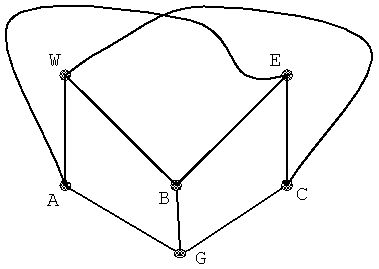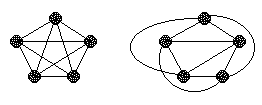# 3 Utilities Puzzle

Years ago, Henry Earnst Dudeney - probably the greatest English puzzlist - wrote:

There are some half-dozen puzzles, as old as the hills, that are perpetually cropping up, and there is hardly a month in the year that does not bring inquiries as to their solution. Occasionally one of these, that one had thought was an extinct volcano, bursts into eruption in a surprising manner. I have received an extraordinary number of letters respecting the ancient puzzle that I have called "Water, Gas and Electricity". It is much older than electric lighting, or even gas, but the new dress brings it up to date. The puzzle is to lay on water, gas, and electricity, from W, G and E, to each of the three houses, A, B and C, without any pipe crossing another.I, too, receive inquiries on a regular basis. Ensuing discussions reveal two distinct mindsets and two distinct approaches to the puzzle. Some people see in it a construction site question of sorts. Obviously no real estate developer was ever concerned (beyond manageable logistics) with hooking up several houses to a required number of utilities. Most of the people, however, feel that such an interpretation voids the puzzle of all mystery and surprise. They grasp intuitively the unannunciated intention of the puzzle and abstract it, even if unwittingly, into the framework of graph theory, similarly to the manner in which Euler handled the Königsberg bridges puzzle. They place 2 sets of three dots opposite each other thinking of each dot as either a utility installation or a house and try to connect the dots without crossings. They fail. For, if the implied question concerns a bipartite graph, then, as it follows from the elementary graph theory, the puzzle has no solution. (A small portion of the latter group are willing to consider a pipe running through a house as nothing in the formulation precludes two edges crossing on an node.)

A graph is a collection of nodes (also called vertices) and edges each connecting a pair of nodes. To visualize a graph, nodes may be thought of as points in space, plane, or another surface, while edges are represented by curves connecting the nodes. Such modeling of a graph is not unique. The only important characterization of a graph is incidence of nodes and edges. All elements of a graph (model) may be moved or deformed continuously while still representing the same graph - the same collection of nodes and edges. In the diagram at the top of the page, 3 pairs of edges intersect forming what is known as crossings. A slight deformation of the diagram leads to a graph (model) with a single crossing:What is remarkable about this graph is that it is impossible to embed it into the plane so as to avoid crossings altogether. (There is no problem of course when embedding it into a three-dimensional space, or, more interestingly, on the surface of a torus.) Graphs that admit such a crossless embedding into the plane are called planar. The fact we are going to establish shortly simply asserts that our graph is not planar. The customary notation for the graph is K3,3 to indicate that the nodes are split into two sets - of 3 nodes each - and that there is enough edges (9 = 3·3 in all) to connect all nodes of one set with every node of the other.

### Theorem

K3,3 is not planar.

### Proof

The proof is not at all difficult and uses Euler's beautiful formula for planar graphs:

F - E + V = 2,

where V is the number of nodes, or vertices (6 in K3,3), E is the number of edges (9), and F is the number of faces - regions bounded by edges that form a closed path, or walk, with no nodes in their interior. A planar graph with a finite number of nodes may always be embedded into a bounded portion of the plane. By convention then, Euler's formula also accounts for the ever present single unbounded face.

Let Fi denote the number of faces bounded by exactly I edges. (A single edge if it connects a node with itself bounds a face. The number of such faces is F1. Two edges that connect the same nodes also form a face. F2 denotes the number of 2-sided faces. Etc. We may assume that there are no double edges; for, their presence does not affect planarity of the graph.) The fact that each edge is included as part of the boundary of exactly two faces leads to the following formula

2E = F1 + 2F2 + 3F3 + 4F4 + ...

For any bipartite graph,

1. F1 = 0 as there are no loops.
2. F2 = 0 because no two nodes are connected by 2 edges (by the convention we made).
3. F3 = 0. For, a 3-sided face would have one side that connects 2 nodes of the same triple of nodes in contradiction with the definition.
4. Fi = 0, for any odd I (as above.)

Thus we get

2E = 4F4 + 6F6 + 8F8 + 10F10 + ...

which must also hold for K3,3 if we assume it is planar. But, for K3,3, V = 6 and E = 9. From Euler's formula it then would follow that F = 2 - V + E = 2 - 6 + 9 = 5. Now,

F = F1 + F2 + F3 + ...
(or F = F4 + F6 + F8 + ... for K3,3. Check, though, that F8 = F10 = ... = 0).

Hence

18 = 2E = 4F4 + 6F6 + 8F8 + 10F10 + ... > 20

The latter is an obvious nonsense. We must conclude therefore that K3,3 is not planar.

### Remarks

1. A different solution is based on the Jordan Curve Theorem. Consider the closed curve AWBECGA as on the second diagram. If one of the curves AE, WC, or BG intersects one of the sides edges AW, WB, BE, EC, CG, or GA, there is nothing to prove. Otherwise, the curve AE lies entirely either inside or outside of AWBECGA. The same is true of the curves WC and BG. Which means that of the three curves at least two lie entirely either inside or outside of AWBECGA. These two curves are bound to intersect.

2. Very much the same proof as above shows that the complete graph (i.e., a graph in which there is an edge for every pair of nodes) with 5 nodes - K5 - is also non-planar. (A proof can be found by evaluating the crossing number of the two graphs.)Those two graphs, K5 and K3,3, are in fact prototypical non-planar graphs. It was shown by the Polish mathematician Kazimierz Kuratowski (1896-1980) that a graph is planar iff it contains neither K5, nor K3,3 as a subgraph.

3. Stuart Anderson came with an idea of embedding K3,3 into a Möbius band whose nonorientability highlights non-planarity of the graph:

I was just looking at the bipartite graph page, and I noticed a different way to see that K3,3 is nonplanar. (No doubt there are many ways to see this.) If the edges AE and WC are connected with straight lines instead of the curves in the figure, you can visualize the graph as a Möbius strip in 3d. Rectangle WABG is a section of the strip, BGEC is the next section along the strip, and ECWA is a third section connecting the first two and completing the strip. Where edges WC and AE cross, we are seeing the half twist in the section ECWA, looking at the strip edge on. Once you see the graph this way, it is obvious how to draw it on a Möbius strip. Just draw three lines across the width of the strip and a line around the edge. The points of intersection are the vertices of K3,3 and the line segments connecting them are the edges. But if K3,3 were planar, it would have a natural orientation inherited from the plane, hence could not be filled with a nonorientable surface. (If K3,3 were imbedded in the plane, the path around the Möbius strip would now appear to follow a patchwork of orientable areas, and so would not reverse orientation.) Therefore K3,3 is nonplanar.

I think this is interesting, because the other two dimensional embedding mentioned (in a torus) can't be used so directly to prove K3,3 is nonplanar, because the torus is orientable. Perhaps other topological properties of the torus could be used instead, but none is as obviously incompatible with a plane embedding as nonorientability.

The same trick works for K5 as well: Taking the vertices 4 at a time you can see trapezoidal strips that you can think of as segments of a Möbius strip. Follow each strip to the edge and imagine that the paper folds under and connects to the next trapezoid. After going around 5 times, you have of course a Möbius strip because you've made an odd number of folds. To draw this graph on a Möbius strip, place 5 equally spaced points along its edge and connect them across the strip with a zigzag line. This way of folding a Möbius strip is very closely related to the cut-the-knot logo, omitting the extra knots at the corners.

Isn't it interesting that the cut-the-knot logo can be used to prove K5 is nonplanar?

### References

1. M. Aigner, G. M. Ziegler, Proofs From The Book, Springer, 2000
2. M. Gardner, The Second Scientific Ameican Book of Mathematical Puzzles and Diversions, The University of Chicago Press, 1987
3. O. Ore, Graphs And Their Uses, MAA 1990
4. I. Stewart, Concepts of Modern Mathematics, Dover, 1995
5. R. J. Trudeau, Introduction to Graph Theory, Dover, 1993
6. D. Wells, The Penguin Book of Curious and Interesting Puzzles, Penguin Books, 1992